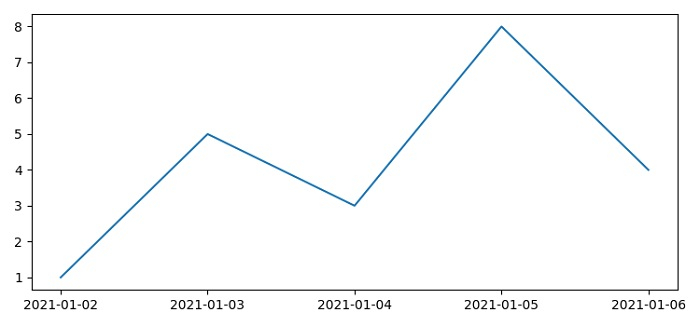# How to plot data against specific dates on the X-axis using Matplotlib?

To plot data against specific dates on the X-axis using matplotlib, we can take the following steps −

• Set the figure size and adjust the padding between and around the subplots.

• Make a list of dates and convert them in datetime format as x.

• Make a list of y data points.

• Set the formatter of the major ticker.

• Set the locator of the major ticker.

• Plot x and y data points using plot() method.

• To display the figure, use show() method.

## Example

from datetime import datetime
from matplotlib import pyplot as plt, dates as mdates

plt.rcParams["figure.figsize"] = [7.50, 3.50]
plt.rcParams["figure.autolayout"] = True

dates = ["01/02/2021", "01/03/2021", "01/04/2021", "01/05/2021", "01/06/2021", ]

x = [datetime.strptime(d, "%m/%d/%Y").date() for d in dates]
y = [1, 5, 3, 8, 4]

ax = plt.gca()
ax.xaxis.set_major_formatter(mdates.DateFormatter("%Y-%m-%d"))
ax.xaxis.set_major_locator(mdates.DayLocator())
ax.plot(x, y)

plt.show()

## Output